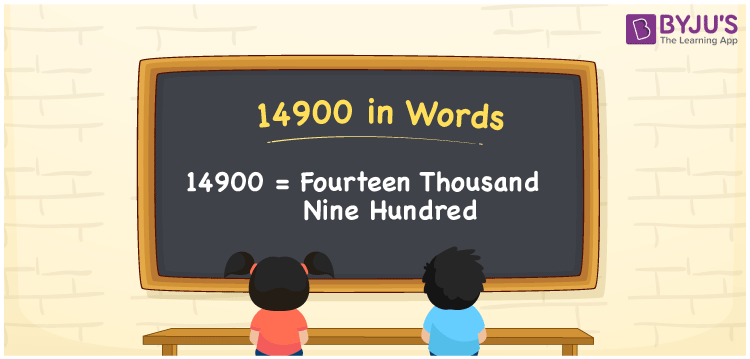# 14900 in Words

14900 in words can be written as Fourteen Thousand Nine Hundred. This article will provide you with an in-depth knowledge of writing numbers in words. For example, if you buy a mobile for Rs. 14900 from the shop, then you can say that “I bought a mobile for Fourteen Thousand Nine Hundred Rupees from the shop”. Get to know more about the numbers in words concept by referring to this article. So, 14900 can be read as “Fourteen Thousand Nine Hundred” in words.

 14900 in words Fourteen Thousand Nine Hundred Fourteen Thousand Nine Hundred in Numbers 14900

## 14900 in English Words## How to Write 14900 in Words?

This section will give students a clear idea of the place value of 14900. It is given as a table below showing the place value of the five digits.

 Ten-Thousands Thousands Hundreds Tens Ones 1 4 9 0 0

The expanded form of 14900 is given here:

1 x Ten Thousands + 4 x Thousands + 9 x Hundreds + 0 × Ten + 0 × One

= 1 x 10000 + 4 x 1000 + 9 x 100

= 10000 + 4000 + 900

= 14900

= Fourteen Thousand Nine Hundred

Hence, 14900 in words is written as Fourteen Thousand Nine Hundred.

14900 is a natural number that precedes 14901 and succeeds 14899.

14900 in words – Fourteen Thousand Nine Hundred

Is 14900 an odd number? – No

Is 14900 an even number? – Yes

Is 14900 a perfect square number? – No

Is 14900 a perfect cube number? – No

Is 14900 a prime number? – No

Is 14900 a composite number? – Yes

## Frequently Asked Questions on 14900 in Words

Q1

### How do you write 14900 in words?

14900 can be written as “Fourteen Thousand Nine Hundred” in words.
Q2

### What is the value of 15000 minus 100?

The value of 15000 minus 100 is 14900. Therefore, 14900 in words is Fourteen Thousand Nine Hundred.
Q3

### Is 14900 a perfect square number?

No, 14900 is not a perfect square number as it is not the product of two similar numbers.
Q4

### Is 14,900 a composite number?

Yes, 14,900 is a composite number as it has more than two factors.
Q5

### What is the value of 14,850 plus 50?

The value of 14,850 plus 50 is 14,900. Therefore, 14,900 in words is Fourteen Thousand Nine hundred.